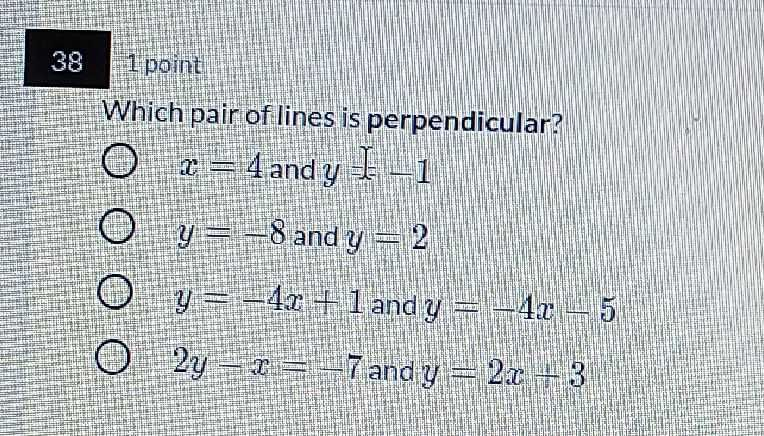### ¿Todavía tienes preguntas de matemáticas?

Pregunte a nuestros tutores expertos
Algebra
Pregunta$$38$$ 1point Which pair of lines is perpendicular?

$$x = 4$$ and $$y = - 1$$

$$y = - 8$$ and $$y = 2$$

$$y = - 4 x + 1$$ and $$y = - 4 x - 5$$

$$2 y - x = - 7$$ and $$y = 2 x + 3$$